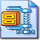## Setting up, editing, formatting and using worksheets

##### Disco Task (2012 new version*)

Skills covered:

• enter data
• widen columns
• text formatting
• wrap text
• merge cells
• rename tabs
• delete worksheets
• insert worksheet
• copy worksheet
• multiplication formula
• SUM formula
• format as currency
• insert rows
• absolute cell reference
• insert footer
• borders
• cell alignment
• format date
• find and replace
• Undo
• format painter
• sort data

* The 2003 task is the old version. The 2007 task has been rewritten and updated.

##### Burgers Task (2012 brand new version*)

Skills covered:

• rename tabs
• delete worksheet
• column widths
• text formatting
• SUM, AVERAGE, MIN, MAX formula
• replicate formula
• cell formatting - colours, borders, underline
• insert rows
• merge and centre
• decimal places
• select non adjacent columns
• column chart
• change chart style
• change chart colour
• add titles, axis labels
• move, resize chart
• undo, redo

* this is NOT the same as the 2003 version of the burgers task. It is a completely new task.

##### Teddy Tots Greetings Cards Task

Skills covered:

• Create a structure and layout within a spreadsheet application
• Enter a list of prices into a spreadsheet
• Write simple formula with a single operator
• Write a SUM function using a cell range
• Format data to currency
• Use appropriate number of decimal places
• Write a formula to calculate a percentage
• Ensure work fits on one A4 sheet
• Set gridlines to show on printing
• Set row and column headers to show on printing
• Show formulas

(Excel 2010)

(Excel 2007)

(Excel 2003)

##### Car Sales

Skills covered:

• Write a SUM formula
• Write a formula to calculate percentages
• Format cells as a percentage
• Format cells to 1 decimal place
• Write and use an absolute cell reference
• Replicate formulae
• Write a formula to calculate the differences between to different percentages
• Ensure work fits on one A4 sheet
• Add name to footer
• Set gridlines to show on printing
• Set row and column headers to show on printing
• Show formulas

Skills covered:

• Setting up a basic spreadsheet
• Basic formulae
• Basic functions
• Basic formatting
• Format painter
• Formatting currency
• Merging cells
• Adding and removing toolbars
• Freezing panes
• Hiding a column
• Creating a copy of the workbook
• Switching between windows
• Saving as a template
• IF formula
• Column chart
• Chart formatting
• Setting printing options
• Find and replace tool
• Sorting data in a table

Skills covered:

• Setting up a spreadsheet
• Basic formula and functions
• Formatting a spreadsheet
• Inserting rows
• Borders
• Headers and footers
• Printing options
• Sorting data
• Hiding a column

##### Supermarket Comparisons Task

Skills covered:

• Setting up a spreadsheet
• Basic formula and functions
• Formatting a spreadsheet
• Resizing columns
• Footers

Suitable for all versions

##### Groovy Music

Skills covered:

• merge cells
• wrap text
• align titles
• basic formulae
• basic functions
• formatting cells
• borders
• gridlines
• sort data
• auto filter
• footers
• hiding cells
• freeze pane
• print area
• show formulas
• IF formula
• VLOOKUP
• charts
• absolute cell reference

Suitable for all versions

##### Online auctions

This spreadsheet exercise provides students with an opportunity to practise:

• adjusting column widths
• basic formatting and cell alignment
• inserting new columns and rows
• MIN, MAX and AVERAGE formulae

Extension tasks are provided

Contributed by Jon Bridgeman of Finham Park School

A test which should take most of an ICT lesson.

A good way to check the level of your students' skills

test instructions

test marksheetSuitable for all versions